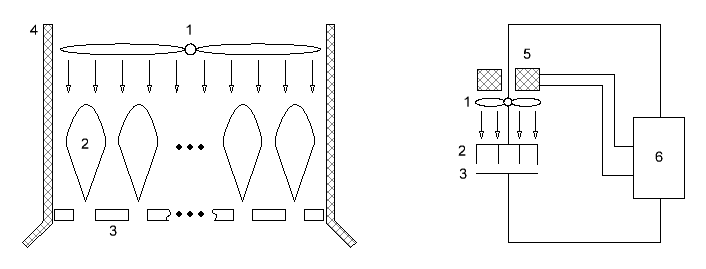Research website of Vyacheslav Gorchilin
2016-08-25
Air motor And diode
This principle available for joint patenting
In this work describes the design and calculation of efficiency And diode. It uses a cylindrical condenser, in which the inner electrode has a needle-shaped surface. In prestavleniem below the device uses the same principle, only plate And diodes are deployed in the plane, and the needle are teardrop-shaped for better flow of the air stream. The ionic wind velocity amplified air pump, thereby achieving a higher efficiency And the diode, and the device turns into a ramjet air engine.
The figure below shows the device (left) and its connection scheme (right). The figure shows:
• 1 — vane air pump;
• 2 teardrop — shaped needle (the first electrode And diodes);
• 3 — the grid plate (the second electrode And the diode);
• 4 — motor housing;
• 5 the electromagnets of the motor of the air pump;
• 6 control unit the pumped diode air pump.The device is simple. Control unit pump (6) creates optimal conditions for the occurrence of the ion wind And the diode (2, 3). Flow from the air pump (1, 5) increases the speed of the ions in the diode, and at the same time — and the General thrust of the engine. Thus, the higher ionic wind velocity, the higher the efficiency of the entire installation. The limit of this strengthening is restricted to the aerodynamic properties of the medium and the cubic dependence of the flow rate of the pump from its power. Limit the speed to develop this engine, apparently, and will be cruising speed for the entire structure.
Rate the increase in efficiency due to the increase of the speed of the ion flow. To do this, take of the above work, the formula (3.10) to increase the efficiency of the second kind: $K_{\eta2} = \frac23 {\mu\,R\,Q_0 \over h^2}$ In this structure, as $$R$$ may be the resistance of the windings of the motor (5), or fuel Converter, which is included in the unit (6). Then from the formula it is possible to allocate a certain average velocity of the ions: $K_{\eta2} = \frac23 \bar V {\,R\,C \over h}, \qquad \bar V = {\mu \, U_0 \over h}$ In our device, this average speed is enhanced by the air flow from the pump. Then the velocity of the ions and this thread's stack: $K_{\eta2} = \frac23 (\bar V + V_{m}) {\,R\,C \over h}$ where $$V_{m}$$ is the velocity of the air flow from the pump. This speed has a dependency, as the cube root of the pump power, so when it is gradual increase, after a significant overall growth of $$K_{\eta2}$$, it will first slow down and then begin to decrease. This maximum and should be achieved. It depends on many factors specific to the design of the device.